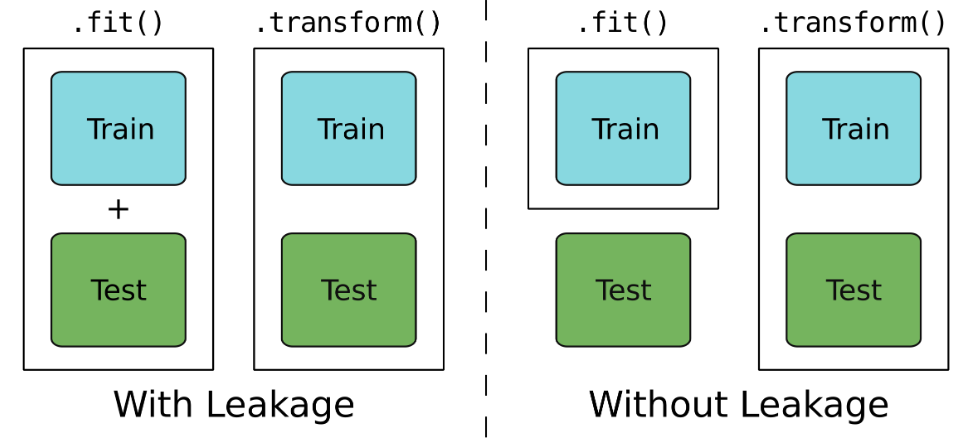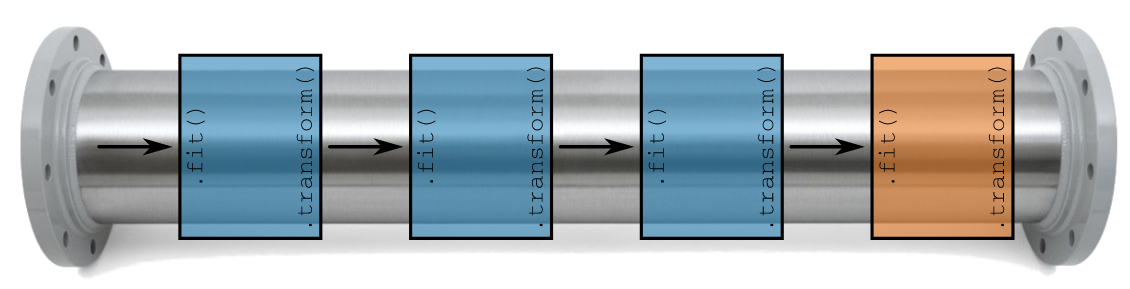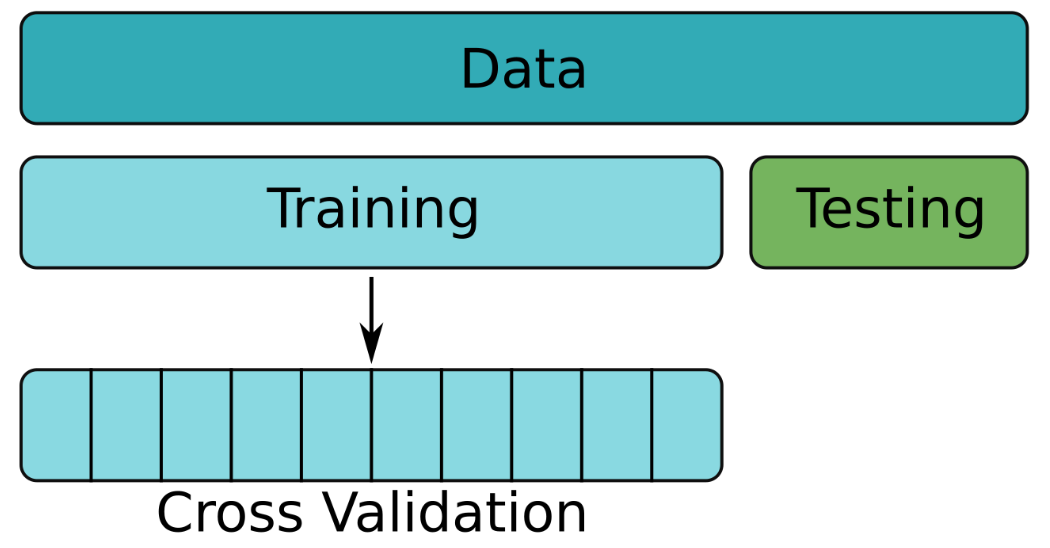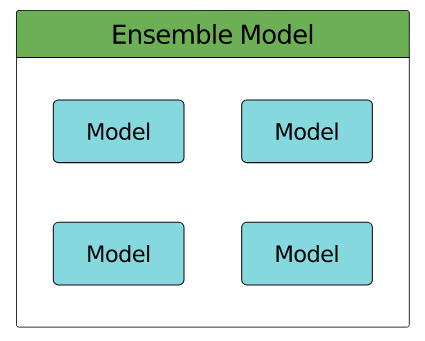import pyspark
from pyspark.sql import SparkSession

import pandas as pd
import numpy as np


## Pipeline

• A watertight model• If test data is included while training, the model will be no longer for objective (leakage)
• Pipeline### Flight duration model - Pipeline stages

You're going to create the stages for the flights duration model pipeline. You will use these in the next exercise to build a pipeline and to create a regression model.

spark = SparkSession.builder.master('local[*]').appName('flights').getOrCreate()

# Read data from CSV file
nullValue='NA')

# Get number of records
print("The data contain %d records." % flights.count())

# View the first five records
flights.show(5)

# Check column data types
print(flights.printSchema())
print(flights.dtypes)

The data contain 275000 records.
+---+---+---+-------+------+---+----+------+--------+-----+
|mon|dom|dow|carrier|flight|org|mile|depart|duration|delay|
+---+---+---+-------+------+---+----+------+--------+-----+
| 10| 10|  1|     OO|  5836|ORD| 157|  8.18|      51|   27|
|  1|  4|  1|     OO|  5866|ORD| 466|  15.5|     102| null|
| 11| 22|  1|     OO|  6016|ORD| 738|  7.17|     127|  -19|
|  2| 14|  5|     B6|   199|JFK|2248| 21.17|     365|   60|
|  5| 25|  3|     WN|  1675|SJC| 386| 12.92|      85|   22|
+---+---+---+-------+------+---+----+------+--------+-----+
only showing top 5 rows

root
|-- mon: integer (nullable = true)
|-- dom: integer (nullable = true)
|-- dow: integer (nullable = true)
|-- carrier: string (nullable = true)
|-- flight: integer (nullable = true)
|-- org: string (nullable = true)
|-- mile: integer (nullable = true)
|-- depart: double (nullable = true)
|-- duration: integer (nullable = true)
|-- delay: integer (nullable = true)

None
[('mon', 'int'), ('dom', 'int'), ('dow', 'int'), ('carrier', 'string'), ('flight', 'int'), ('org', 'string'), ('mile', 'int'), ('depart', 'double'), ('duration', 'int'), ('delay', 'int')]

from pyspark.sql.functions import round

# Convert 'mile' to 'km' and drop 'mile' column
flights = flights.withColumn('km', round(flights.mile * 1.60934, 0)).drop('mile')

from pyspark.ml.feature import StringIndexer, OneHotEncoder, VectorAssembler
from pyspark.ml.regression import LinearRegression

# Convert categorical strings to index values
indexer = StringIndexer(inputCol='org', outputCol='org_idx')

# One-hot encode index values
onehot = OneHotEncoder(
inputCols=['org_idx', 'dow'],
outputCols=['org_dummy', 'dow_dummy']
)

# Assemble predictors into a single column
assembler = VectorAssembler(inputCols=['km', 'org_dummy', 'dow_dummy'], outputCol='features')

# A linear regression object
regression = LinearRegression(labelCol='duration')


### Flight duration model: Pipeline model

You're now ready to put those stages together in a pipeline.

You'll construct the pipeline and then train the pipeline on the training data. This will apply each of the individual stages in the pipeline to the training data in turn. None of the stages will be exposed to the testing data at all: there will be no leakage!

Once the entire pipeline has been trained it will then be used to make predictions on the testing data.

from pyspark.ml import Pipeline

flights_train, flights_test = flights.randomSplit([0.8, 0.2])

# Construct a pipeline
pipeline = Pipeline(stages=[indexer, onehot, assembler, regression])

# Train the pipeline on the training data
pipeline = pipeline.fit(flights_train)

# Make predictions on the test data
predictions = pipeline.transform(flights_test)


### SMS spam pipeline

You haven't looked at the SMS data for quite a while. Last time we did the following:

• split the text into tokens
• removed stop words
• applied the hashing trick
• converted the data from counts to IDF and
• trained a linear regression model.

Each of these steps was done independently. This seems like a great application for a pipeline!

from pyspark.sql.types import StructType, StructField, IntegerType, StringType

# Specify column names and types
schema = StructType([
StructField("id", IntegerType()),
StructField("text", StringType()),
StructField("label", IntegerType())
])

# Read data from CSV file

sms.show(5)

+---+--------------------+-----+
| id|                text|label|
+---+--------------------+-----+
|  1|Sorry, I'll call ...|    0|
|  2|Dont worry. I gue...|    0|
|  3|Call FREEPHONE 08...|    1|
|  4|Win a 1000 cash p...|    1|
|  5|Go until jurong p...|    0|
+---+--------------------+-----+
only showing top 5 rows


from pyspark.ml.feature import Tokenizer, StopWordsRemover, HashingTF, IDF
from pyspark.ml.classification import LogisticRegression

# Break text into tokens at non-word characters
tokenizer = Tokenizer(inputCol='text', outputCol='words')

# Remove stop words
remover = StopWordsRemover(inputCol=tokenizer.getOutputCol(), outputCol='terms')

# Apply the hashing trick and transform to TF-IDF
hasher = HashingTF(inputCol=remover.getOutputCol(), outputCol='hash')
idf = IDF(inputCol=hasher.getOutputCol(), outputCol='features')

# Create a logistic regression object and add everything to a pipeline
logistic = LogisticRegression()
pipeline = Pipeline(stages=[tokenizer, remover, hasher, idf, logistic])


## Cross-Validation### Cross validating simple flight duration model

You've already built a few models for predicting flight duration and evaluated them with a simple train/test split. However, cross-validation provides a much better way to evaluate model performance.

In this exercise you're going to train a simple model for flight duration using cross-validation. Travel time is usually strongly correlated with distance, so using the km column alone should give a decent model.

assembler = VectorAssembler(inputCols=['km'], outputCol='features')

flights = assembler.transform(flights.drop('features'))

flights.show(5)

+---+---+---+-------+------+---+------+--------+-----+------+--------+
|mon|dom|dow|carrier|flight|org|depart|duration|delay|    km|features|
+---+---+---+-------+------+---+------+--------+-----+------+--------+
| 10| 10|  1|     OO|  5836|ORD|  8.18|      51|   27| 253.0| [253.0]|
|  1|  4|  1|     OO|  5866|ORD|  15.5|     102| null| 750.0| [750.0]|
| 11| 22|  1|     OO|  6016|ORD|  7.17|     127|  -19|1188.0|[1188.0]|
|  2| 14|  5|     B6|   199|JFK| 21.17|     365|   60|3618.0|[3618.0]|
|  5| 25|  3|     WN|  1675|SJC| 12.92|      85|   22| 621.0| [621.0]|
+---+---+---+-------+------+---+------+--------+-----+------+--------+
only showing top 5 rows


from pyspark.ml.tuning import ParamGridBuilder, CrossValidator
from pyspark.ml.evaluation import RegressionEvaluator

flights_train, flights_test = flights.randomSplit([0.8, 0.2])

# Create an empty parameter grid
params = ParamGridBuilder().build()

# Create objects for building and evaluating a regression model
regression = LinearRegression(labelCol='duration')
evaluator = RegressionEvaluator(labelCol='duration')

# Create a cross validator
cv = CrossValidator(estimator=regression, estimatorParamMaps=params,
evaluator=evaluator, numFolds=5)

# Train and test model on multiple folds of the training data
cv = cv.fit(flights_train)


### Cross validating flight duration model pipeline

The cross-validated model that you just built was simple, using km alone to predict duration.

Another important predictor of flight duration is the origin airport. Flights generally take longer to get into the air from busy airports. Let's see if adding this predictor improves the model!

In this exercise you'll add the org field to the model. However, since org is categorical, there's more work to be done before it can be included: it must first be transformed to an index and then one-hot encoded before being assembled with km and used to build the regression model. We'll wrap these operations up in a pipeline.

params = ParamGridBuilder().build()

# Create regression model
regression = LinearRegression(labelCol='duration')
evaluator = RegressionEvaluator(labelCol='duration')

# Create an indexer for the org field
indexer = StringIndexer(inputCol='org', outputCol='org_idx')

# Create an one-hot encoder for the indexed org field
onehot = OneHotEncoder(inputCol='org_idx', outputCol='org_dummy')

# Assemble the km and one-hot encoded fields
assembler = VectorAssembler(inputCols=['km', 'org_dummy'], outputCol='features')

# Create a pipeline and cross-validator
pipeline = Pipeline(stages=[indexer, onehot, assembler, regression])
cv = CrossValidator(estimator=pipeline,
estimatorParamMaps=params,
evaluator=evaluator)


### Optimizing flights linear regression

Up until now you've been using the default hyper-parameters when building your models. In this exercise you'll use cross validation to choose an optimal (or close to optimal) set of model hyper-parameters.

params = ParamGridBuilder()

# Add grids for two parameters
params = params.addGrid(regression.regParam, [0.01, 0.1, 1.0, 10.0])\

# Build the parameter grid
params = params.build()
print('Number of models to be tested: ', len(params))

# Create cross-validator
cv = CrossValidator(estimator=pipeline, estimatorParamMaps=params,
evaluator=evaluator, numFolds=5)

Number of models to be tested:  12


### Dissecting the best flight duration model

You just set up a CrossValidator to find good parameters for the linear regression model predicting flight duration.

Now you're going to take a closer look at the resulting model, split out the stages and use it to make predictions on the testing data.

flights_train, flights_test = flights.drop('features').randomSplit([0.8, 0.2])

# Train the data
cvModel = cv.fit(flights_train)

best_model = cvModel.bestModel

# Look at the stages in the best model
print(best_model.stages)

# Get the parameters for the LinearRegression object in the best model
best_model.stages.extractParamMap()

# Generate predictions on test data using the best model then calculate RMSE
predictions = best_model.transform(flights_test)
evaluator.evaluate(predictions)

[StringIndexerModel: uid=StringIndexer_e6270b361418, handleInvalid=error, OneHotEncoderModel: uid=OneHotEncoder_f0cb230aef6c, dropLast=true, handleInvalid=error, VectorAssembler_7f7413ad3499, LinearRegressionModel: uid=LinearRegression_61f3d4b255f6, numFeatures=8]

11.217337853713733

### SMS spam optimized

The pipeline you built earlier for the SMS spam model used the default parameters for all of the elements in the pipeline. It's very unlikely that these parameters will give a particularly good model though.

In this exercise you'll set up a parameter grid which can be used with cross validation to choose a good set of parameters for the SMS spam classifier.

params = ParamGridBuilder()

# Add grid for hashing trick parameters
params = params.addGrid(hasher.numFeatures, (1024, 4096, 16384))\

# Add grid for logistic regression parameters
params = params.addGrid(logistic.regParam, (0.01, 0.1, 1.0, 10.0))\

# Build parameter grid
params = params.build()

print('Number of models to be tested: ', len(params))

Number of models to be tested:  72


## Ensemble• collection of models
• Wisdom of the Crowd - collective opinion of a group better than that of a single expert
• Random Forest
• an ensemble of Decision Tree
• Creating model diversity
• each tree trained on random subset of data
• random subset of features used for splitting at each node
• No two trees in the forest should be the same
• Iterative boosting algorithm:
1. Build a Decision Tree and add to ensemble
2. Predict label for each training instance using ensemble
3. Compare predictions with known labels
4. Emphasize training instances with incorrect predictions

### Delayed flights with Gradient-Boosted Trees

You've previously built a classifier for flights likely to be delayed using a Decision Tree. In this exercise you'll compare a Decision Tree model to a Gradient-Boosted Trees model.

from pyspark.ml.classification import RandomForestClassifier, GBTClassifier
from pyspark.ml.evaluation import BinaryClassificationEvaluator

assembler = VectorAssembler(inputCols=['mon', 'depart', 'duration'], outputCol='features')
flights = assembler.transform(flights.drop('features'))
flights = flights.withColumn('label', (flights.delay >= 15).cast('integer'))
flights = flights.select('mon', 'depart', 'duration', 'features', 'label')
flights = flights.dropna()

flights.show(5)

+---+------+--------+-----------------+-----+
|mon|depart|duration|         features|label|
+---+------+--------+-----------------+-----+
| 10|  8.18|      51| [10.0,8.18,51.0]|    1|
| 11|  7.17|     127|[11.0,7.17,127.0]|    0|
|  2| 21.17|     365|[2.0,21.17,365.0]|    1|
|  5| 12.92|      85| [5.0,12.92,85.0]|    1|
|  3| 13.33|     182|[3.0,13.33,182.0]|    1|
+---+------+--------+-----------------+-----+
only showing top 5 rows


from pyspark.ml.classification import DecisionTreeClassifier, GBTClassifier
from pyspark.ml.evaluation import BinaryClassificationEvaluator
from pprint import pprint

flights_train, flights_test = flights.randomSplit([0.8, 0.2])

# Create model objects and train on training data
tree = DecisionTreeClassifier().fit(flights_train)
gbt = GBTClassifier().fit(flights_train)

# Compare AUC on test data
evaluator = BinaryClassificationEvaluator()
evaluator.evaluate(tree.transform(flights_test))
evaluator.evaluate(gbt.transform(flights_test))

# Find the number of trees and the relative importance of features
pprint(gbt.trees)
print(gbt.featureImportances)

[DecisionTreeRegressionModel: uid=dtr_ec0910e25dea, depth=5, numNodes=63, numFeatures=3,
DecisionTreeRegressionModel: uid=dtr_fca176dc37a4, depth=5, numNodes=63, numFeatures=3,
DecisionTreeRegressionModel: uid=dtr_ff05809a5d0d, depth=5, numNodes=63, numFeatures=3,
DecisionTreeRegressionModel: uid=dtr_c0178826c7f2, depth=5, numNodes=63, numFeatures=3,
DecisionTreeRegressionModel: uid=dtr_e3d7c7cf801d, depth=5, numNodes=63, numFeatures=3,
DecisionTreeRegressionModel: uid=dtr_b28386703df3, depth=5, numNodes=63, numFeatures=3,
DecisionTreeRegressionModel: uid=dtr_236a1b0a44cc, depth=5, numNodes=63, numFeatures=3,
DecisionTreeRegressionModel: uid=dtr_5702ac9781e9, depth=5, numNodes=63, numFeatures=3,
DecisionTreeRegressionModel: uid=dtr_1294cc0afc51, depth=5, numNodes=63, numFeatures=3,
DecisionTreeRegressionModel: uid=dtr_9c3a2c2f669c, depth=5, numNodes=63, numFeatures=3,
DecisionTreeRegressionModel: uid=dtr_ba4aef54784c, depth=5, numNodes=63, numFeatures=3,
DecisionTreeRegressionModel: uid=dtr_760d589922ac, depth=5, numNodes=63, numFeatures=3,
DecisionTreeRegressionModel: uid=dtr_6d07166b635b, depth=5, numNodes=63, numFeatures=3,
DecisionTreeRegressionModel: uid=dtr_c807be867213, depth=5, numNodes=63, numFeatures=3,
DecisionTreeRegressionModel: uid=dtr_ef74f5e2c3b4, depth=5, numNodes=63, numFeatures=3,
DecisionTreeRegressionModel: uid=dtr_858147b5b20b, depth=5, numNodes=63, numFeatures=3,
DecisionTreeRegressionModel: uid=dtr_f0ff74220975, depth=5, numNodes=63, numFeatures=3,
DecisionTreeRegressionModel: uid=dtr_958292546340, depth=5, numNodes=63, numFeatures=3,
DecisionTreeRegressionModel: uid=dtr_e387146dd7b3, depth=5, numNodes=63, numFeatures=3,
DecisionTreeRegressionModel: uid=dtr_1d883b4c0434, depth=5, numNodes=63, numFeatures=3]
(3,[0,1,2],[0.3996945565930914,0.3279675109854171,0.27233793242149146])


### Delayed flights with a Random Forest

In this exercise you'll bring together cross validation and ensemble methods. You'll be training a Random Forest classifier to predict delayed flights, using cross validation to choose the best values for model parameters.

You'll find good values for the following parameters:

• featureSubsetStrategy — the number of features to consider for splitting at each node and
• maxDepth — the maximum number of splits along any branch.
from pyspark.ml.classification import RandomForestClassifier

# Create a random forest classifier
forest = RandomForestClassifier()

# Create a parameter grid
params = ParamGridBuilder() \
.addGrid(forest.featureSubsetStrategy, ['all', 'onethird', 'sqrt', 'log2']) \
.build()

# Create a binary classification evaluator
evaluator = BinaryClassificationEvaluator()

# Create a cross-validator
cv = CrossValidator(estimator=forest, estimatorParamMaps=params,
evaluator=evaluator, numFolds=5)


### Evaluating Random Forest

In this final exercise you'll be evaluating the results of cross-validation on a Random Forest model.

cvModel = cv.fit(flights_train)

# Average AUC for each parameter combination in grid
avg_auc = cvModel.avgMetrics

# Average AUC for the best model
best_model_auc = max(avg_auc)

# What's the optimal paramter value?
opt_max_depth = cvModel.bestModel.explainParam('maxDepth')
opt_feat_substrat = cvModel.bestModel.explainParam('featureSubsetStrategy')

# AUC for best model on test data
best_auc = evaluator.evaluate(cvModel.transform(flights_test))
print(best_auc)

0.6844690961353807